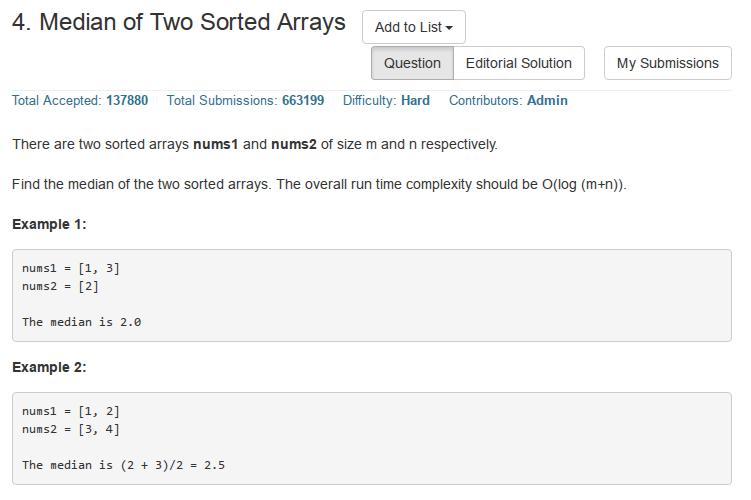﻿ 4. Median of Two Sorted Arrays

### Median of Two Sorted Arrays### Solution

#include <iostream>
#include <string>
#include <algorithm>
#include <vector>
using namespace std;
class Solution
{
public:
double findMedianSortedArrays(vector<int>& nums1, vector<int>& nums2)
{
int m = nums1.size(),n = nums2.size();
if((m + n) % 2 == 0)
return (FindKth(nums1,nums2,(m + n) / 2) + FindKth(nums1,nums2,(m + n) / 2 + 1))  / 2.0;
else
return FindKth(nums1,nums2,(m + n + 1) / 2);
}
//找到第k个最大的数,K应小于等于m+n,这里未做验证
int FindKth(vector<int> &M,vector<int>& N,int K)
{
int m = M.size(),n = N.size();
//设置M为较短的数组
if(m > n) return FindKth(N,M,K);
//边界条件:注意下面两行不能互换,因为可能在K == 1时,较短的数组M可能为空
//比如M=[1,2] N=[3,4]
if(m == 0) return  N[K - 1];
if(K == 1) return min(M,N);
//M和N的指针分别设置为pm,pn,使得pm+pn=K
int pm = min(K / 2,m);
int pn = K - pm;
//如果M上的一段必定位于前K个数中
if(M[pm - 1] < N[pn - 1])
{
vector<int> vec(M.begin() + pm,M.end());
return FindKth(vec,N,K - pm);
}
//否则在N上的一段必然属于前K个数中
else
{
vector<int> vec(N.begin() + pn,N.end());
return FindKth(M,vec,K - pn);
}
}
//调试函数
void PrintVector(vector<int> vec)
{
for(vector<int>::iterator pos = vec.begin(); pos != vec.end(); ++pos)
if(pos + 1 == vec.end()) cout<<*pos<<endl;
else cout<<*pos<<"  ";
}
};
//=======================Driver===========================
int main()
{
int M[] = {1,2};
int N[] = {3,4};
vector<int> nums1(M,M + sizeof(M) / sizeof(int));
vector<int> nums2(N,N + sizeof(N) / sizeof(int));
Solution s;
cout<<s.findMedianSortedArrays(nums1,nums2)<<endl;
//cout<<s.SearchPos(nums1,2);
return 0;
}

/*
===========complexity==============
Time Complexity:O(log(m+n))
Space Complexity:O(1)
===========makefile=================
all:
@g++  solve.cpp -o   solve
@./solve
clean:
rm -f solve
reset
===========leetcode accepted========
2080 / 2080 test cases passed.
Status: Accepted
Runtime: 32 ms
Submitted: 0 minutes ago
===========submission date=========
2017-01-09 Mon 11:03 PM
*/


### Analysis

• 第K个数在递归的时候可能会大于某一个数组的长度,因此要保证某一个数组长度始终为最小(这里选择M),并且, 要选取K/2和m中的最小值,以为K/2也可能超过m.
• K的最小值为1,这是边界条件之一
• 由于短数组不断的减少(我们不断维持第一个位置也就是M为较短的数组,如果它在某事较长,则和N互换),最少减少到0,这是边界条件之二.
• m=0的边界条件应该先于K=1的边界条件进行判断

### Supplement

$KM>KN时$:
• 整体的中位数肯定不在数组M的右半侧,具体范围为$(\lfloor\frac{m+1}{2}\rfloor,m]$
• 整体的中位数也不会在数组N的左半侧,具体范围为$[1,\lfloor\frac{n+1}{2}\rfloor)$

1)$KM>KN时$:
• 整体的中位数肯定不在数组M的右半侧,具体范围为$(\lfloor\frac{m+1}{2}\rfloor,m]$
• 整体的中位数也不会在数组N的左半侧,具体范围为$[1,\lfloor\frac{n+1}{2}\rfloor)$
2)$KM < KN 时$:
• 整体的中位数肯定不在数组M的左半侧,具体范围为$[1,\lfloor\frac{m+1}{2}\rfloor)$
• 整体的中位数也不会在数组N的右半侧,具体范围为$(\lfloor\frac{n+1}{2}\rfloor),n]$
3)$KM == KN时$:
• 当m+n为奇数时,则m和n一奇一偶,这种情况奇数数组分界点左右两侧数字的数目相同, 而偶数数组分界点左侧比右侧少一个数,但是数组M和N合并后,共奇数个数,中位数的值一定等于KM或KN. 因此直接返回KM或者KN即可.
• 当m+n为偶数时,则m和n必然都为偶数或者都为奇数:
1)如果都是奇数,那么返回KM或KN即可.
2)如果都是偶数,由于KM和KN相等,我们不妨设合并后,KM在KN之前, 那么中位数必然是KN和KN右毗连的数之和的二分之一.KN右毗连的数为min{M[im+1],N[in+1]}. 于是此时中位数为(KN+min{M[im+1],N[in+1]})/2.

Reference
1.http://www.lifeincode.net/programming/leetcode-median-of-two-sorted-arrays-more-elegant-solution/
2.http://yucoding.blogspot.jp/2013/01/leetcode-question-50-median-of-two.html